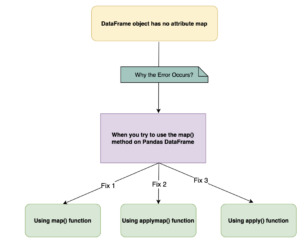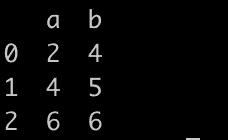# How to Fix AttributeError: ‘DataFrame’ object has no attribute ‘map’Diagram

AttributeError: ‘DataFrame’ object has no attribute ‘map’ error typically occurs when you try to “use the map() method on a Pandas DataFrame.” The map() method is used to apply a function to each element in an iterable, but it is not defined for DataFrames.

Here are three ways to fix the AttributeError: ‘DataFrame’ object has no attribute ‘map’ error.

1. Using the map() function single column
2. Using the applymap() function on an entire DataFrame
3. Using the apply() function on an Axis of the DataFrame

## Reproduce the error

``````import pandas as pd

df = pd.DataFrame({'a': [1, 2, 3], 'b': [4, 5, 6]})

# Apply the map method to the 'a' column
df['a'] = df.map(lambda x: x * 2)

print(df)``````

Output

``````AttributeError: 'DataFrame' object has no attribute 'map'
``````

## How to fix it?

### Solution 1: Using the map() function on DataFrame column

``````import pandas as pd

df = pd.DataFrame({'a': [1, 2, 3], 'b': [4, 5, 6]})

# Apply the map method to the 'a' column
df['a'] = df['a'].map(lambda x: x * 2)

print(df)``````

Output### Solution 2: Using the applymap() function on an Entire DataFrame

``````import pandas as pd

df = pd.DataFrame({'a': [1, 2, 3], 'b': [4, 5, 6]})

# Apply the function to every element in the DataFrame
df = df.applymap(lambda x: x * 2)

print(df)
``````

Output

``````   a   b
0  2   8
1  4  10
2  6  12
``````

### Solution 3: Using the apply() function on an axis of a DataFrame

``````import pandas as pd

df = pd.DataFrame({'a': [1, 2, 3], 'b': [4, 5, 6]})

# Apply the function to each column (axis=0) or row (axis=1)
df = df.apply(lambda x: x * 2, axis=0)

print(df)
``````

Output

``````   a   b
0  2   8
1  4  10
2  6  12 ``````

I hope this helps! Let me know if you have any other questions.

## Related posts

AttributeError: ‘DataFrame’ object has no attribute ‘reshape’

AttributeError: ‘DataFrame’ object has no attribute ‘iteritems’

AttributeError: ‘DataFrame’ object has no attribute ‘data’

AttributeError: ‘DataFrame’ object has no attribute ‘concat’

AttributeError: ‘DataFrame’ object has no attribute ‘ix’

This site uses Akismet to reduce spam. Learn how your comment data is processed.